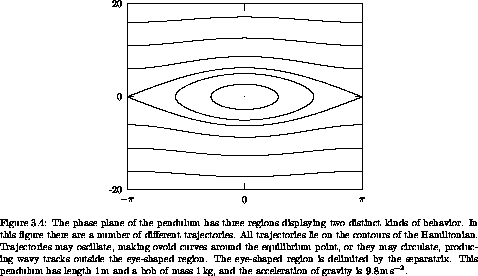## 3.3  One Degree of Freedom

The solutions of time-independent systems with one degree of freedom can be found by quadrature. Such systems conserve the Hamiltonian: the Hamiltonian has a constant value on each realizable trajectory. We can use this constraint to eliminate the momentum in favor of the coordinate. Thus Hamilton's equations reduce to a single equation Dq(t) = f(q(t)). The solution q can be expressed as a definite integral.

A geometric view reveals more structure. Time-independent systems with one degree of freedom have a two-dimensional phase space. Energy is conserved, so all orbits are level curves of the Hamiltonian. The possible orbit types are restricted to curves that are contours of a real-valued function. The possible orbits are paths of constant altitude in the mountain range on the phase plane described by the Hamiltonian.

Only a few characteristic features are possible. There are points that are stable equilibria of the dynamical system. These are the peaks and pits of the Hamiltonian mountain range. These equilibria are stable in the sense that neighboring trajectories on nearby contours stay close to the equilibrium point. There are orbits that trace simple closed curves on contours that surround a peak or pit, or perhaps several peaks. There are also trajectories lying on contours that cross at a saddle point. The crossing point is an unstable equilibrium, unstable in the sense that neighboring trajectories leave the vicinity of the equilibrium point. Such contours that cross at saddle points are called separatrices (singular: separatrix), contours that ``separate'' two regions of distinct behavior.